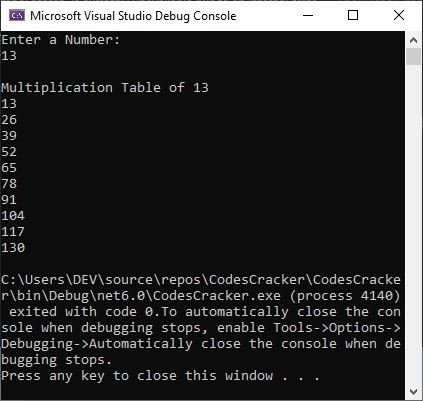# C# Loops with Examples | for, while, and do-while Loop in C#

Loops in C# or in any other programming language are used when we need to execute some block of code for the required number of times. Or we can use a loop to continue executing some defined block of code until the specified condition evaluates to false.

We are going to discuss three types of loops, which are:

## C# while loop

The general form of the while loop in C# is:

```while (condition)
{
// block of code to continue
// its execution until the
// condition evaluates to be false
}```

For example

```Console.WriteLine("Enter a Number: ");

int i = 1;

Console.WriteLine("\nMultiplication Table of {0}", num);
while (i <= 10)
{
Console.WriteLine(num * i);
i++;
}```

The sample run with user input 3 should exactly be as shown in the snapshot given below.The dry run of above C# program should be:

• Using the first line of code, the string or text "Enter a Number: " will be printed on the output console.
• When the user types a value and hits the ENTER key, that value will be initialized to the variable num using the second line of code.
• Using the third line of code, 1 will be initialized to the variable i.
• And using the fourth line of code, a new line will be inserted using \n and then the text "Multiplication Table of 3" (3 is the number entered by user at run-time of the program) will be printed on the output console.
• Now the condition of the while loop will be evaluated.
• Since the condition i <= 10 or 1 <= 10 evaluates to be true. Therefore, program flow goes inside the loop and executes the two written C# statements inside it.
• The first statement prints the value of num * i, which will be 3 * 1 or 3. And using the second statement, the value of i will be incremented by 1. Therefore i = 2 now
• Again the condition of the while loop will be evaluated with new values. That is i < = 10 or 2 <= 10 again evaluates to be true, therefore the program flow again goes inside the loop.
• This process continues, until the condition is evaluated as false.

## C# do...while loop

The do...while loop works similar to the while loop, but instead the block of code inside the loop will be executed at once, even if the defined condition is evaluated to be false at first. Here is the general form of the do...while loop in C#:

```do
{
// block of code to be executed
// at first, and then continue
// its execution until the 'condition'
// evaluates to be false
} while (condition);```

For example:

```int num = 10;
do
{
Console.WriteLine("Inside the do...while loop");
} while (num < 0);```

As you can see from this C# example, the condition num < 0 or 10 < 0 is false at first evaluation. But still, the block of code available inside the do...while loop will be executed. Therefore, the output should exactly be:

`Inside the do...while loop`

Now let me create another example that does the same job as the example used in the while loop:

```Console.WriteLine("Enter a Number: ");

int i = 1;

Console.WriteLine("\nMultiplication Table of {0}", num);
do
{
Console.WriteLine(num * i);
i++;
} while (i <= 10);```

Now, with user input number 7, the sample run of this C# example to show how the do...while loop works should be:

```Enter a Number:
7

Multiplication Table of 7
7
14
21
28
35
42
49
56
63
70```

## C# for loop

Unlike the while and do...while loops, the for loop contains initialization, condition checking, and an update statement all in one place. Here is the general form of the for loop in C#:

```for (initialize; condition; update)
{
// block of code to be executed
// until the "condition" evaluates
// to be false
}```

The initialize statement will be executed once, at the start of the loop. The condition statement will be executed every time before entering the loop. And after executing all the blocks of code defined inside the loop, the update statement will be executed. For example:

```Console.WriteLine("Enter a Number: ");

Console.WriteLine("\nMultiplication Table of {0}", num);
for (int i=1;  i <= 10; i++)
Console.WriteLine(num * i);```

With user input 13, the sample run of this C# example is shown in the snapshot given below.## C# foreach loop

The foreach loop is used when we need to loop through each element of a specified array. Here is the general form of the foreach loop in C#.

```foreach (type x in array)
{
// block of code to be executed
// until the last element of the
// array
}```

For example:

```string[] cities = { "Boston", "Dallas", "Houston", "El Paso", "Phoenix" };
foreach(string x in cities)
{
Console.WriteLine(x);
}```

The output should exactly be:

```Boston
Dallas
Houston
El Paso
Phoenix```

C# Online Test

« Previous Tutorial Next Tutorial »